Courses

# Test: Combinatory- 3

## 15 Questions MCQ Test RRB JE for Computer Science Engineering | Test: Combinatory- 3

Description
This mock test of Test: Combinatory- 3 for Computer Science Engineering (CSE) helps you for every Computer Science Engineering (CSE) entrance exam. This contains 15 Multiple Choice Questions for Computer Science Engineering (CSE) Test: Combinatory- 3 (mcq) to study with solutions a complete question bank. The solved questions answers in this Test: Combinatory- 3 quiz give you a good mix of easy questions and tough questions. Computer Science Engineering (CSE) students definitely take this Test: Combinatory- 3 exercise for a better result in the exam. You can find other Test: Combinatory- 3 extra questions, long questions & short questions for Computer Science Engineering (CSE) on EduRev as well by searching above.
QUESTION: 1

### How many onto (or surjective) functions are there from an n-element (n >= 2) set to a 2-element set?

Solution:

Total possible number of functions is 2n. In mathematics, a function f from a set X to a set Y is surjective (or onto), or a surjection, if every element y in Y has a corresponding element x in X such that f(x) = y (Source: http://en.wikipedia.org/wiki/Surjective_function) There are total 2 functions out of 2n that are NOT onto: one that maps to all 1s and other that maps to all 2s. Therefore total number of onto functions is 2n - 2.

QUESTION: 2

### What is the possible number of reflexive relations on a set of 5 elements?

Solution:

Number of reflexive relations is 2n2-n which is 220 for n = 5

QUESTION: 3

### What is the maximum number of different Boolean functions involving n Boolean variables?

Solution:

No of inputs sequences possible for a n variable Boolean function = 2n Each input sequence can give either T or F as output ( 2 possible values ) So, Total no of Boolean functions are -

2X2X2X2X2X2X.............X2X2X2X2X2X2 <-------------------- 2n Times --------------> 22n

QUESTION: 4

Suppose that a robot is placed on the Cartesian plane. At each step it is allowed to move either one unit up or one unit right, i.e., if it is at (i,j) then it can move to either (i+1,j) or (i,j+1). How many distinct paths are there for the robot to reach the point (10,10) starting from the initial position (0, 0)

Solution:

At each move, robot can move either 1 unit right, or 1 unit up, and there will be 20 such moves required to reach (10,10) from (0,0). So we have to divide these 20 moves, numbered from 1 to 20, into 2 groups: right group and up group. Right group contains those moves in which we move right, and up group contains those moves in which we move up. Each group contains 10 elements each. So basically, we have to divide 20 things into 2 groups of 10 10 things each, i.e., we need to find all possible arrangements of {r, r, r, r, r, r, r, r, r, r, u, u, u, u, u, u, u, u, u, u} where r represents right move and u represents up move. The arrangements can can be done in 20! / (10!∗10!) = 20C10 ways. So option (A) is correct.

QUESTION: 5

Consider the data given in above question. Suppose that the robot is not allowed to traverse the line segment from (4,4) to (5,4). With this constraint, how many distinct paths are there for the robot to reach (10,10) starting from (0,0)?

Solution:

Since we are not allowed to traverse from (4,4) to (5,4), we subtract all those paths which were passing through (4,4) to (5,4). To count number of paths passing through (4,4) to (5,4), we find number of paths from (0,0) to (4,4), and then from (5,4) to (10,10).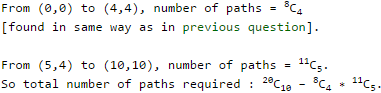So option (D) is correct.

QUESTION: 6

Let ∑ be a finite non-empty alphabet and let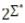be the power set of ∑*. Which one of the following is TRUE?

Solution:
QUESTION: 7

The number of different n × n symmetric matrices with each element being either 0 or 1 is: (Note: power(2, x) is same as 2x)

Solution:

We are considering a symmetric matrix (given in question). So, we need to look at half of elements ie. either upper or lower traingle i.e. A[i][j] = A[j][i] Hence, No. of elements = (n^2 + n)/2 Since, we have only two elements : 0 & 1 No. of choices = 2 Therefore, No. of possibilities = 2 ^ (No. of elements) = 2 ^ ((n^2 + n)/2) = power (2 , (n^2 + n)/2)

QUESTION: 8

Mala has a colouring book in which each English letter is drawn two times. She wants to paint each of these 52 prints with one of k colours, such that the colour-pairs used to colour any two letters are different. Both prints of a letter can also be coloured with the same colour. What is the minimum value of k that satisfies this requirement ?

Solution:

This question is slightly ambiguous. So first let us understand what question is asking. So in a book, we have letters A-Z and each letter is printed twice, so there are 52 letters. Now we have to color each letter, so we need a pair of colors for that, because each letter is printed twice. Also in a pair, both colors can be some. Now condition is that a pair of colors can't be used more than once. So suppose Mala has 3 colors : Red, Blue, Green. She can color as follows : (A,A) : (Red,Red), (B,B) : (Blue,Blue), (C,C) : (Green,Green), (D,D) : (Red,Blue), (E,E) : (Red,Green), (F,F) : (Blue,Green). Now we don't have more pairs of colors left, we have used all pairs, but could color only 6 letters out of 26. So question is to find minimum no. of colors, so that we could color all 26 letters. So if Mala has k colors, she can have k pairs of same colors, thus coloring k letters, then kC2 other pairs of colors, thus coloring kC2 more letters. So total no. of letters colored = k+kC2=k+k(k−1)2=k(k+1)2. So we want k(k+1)2≥26 i.e. k(k+1)≥52, so k≥7, so option (C) is correct.

QUESTION: 9

Let A be a sequence of 8 distinct integers sorted in ascending order. How many distinct pairs of sequences, B and C are there such that
(i) each is sorted in ascending order,
(ii) B has 5 and C has 3 elements, and
(iii) the result of merging B and C gives A?

Solution:

Suppose you have selected 3 elements from 8 in 8C3 ways, the remaining elements are treated as another array and merging both the arrays gives the sorted array. Here, you can select either 3 or 5. => 8C3 = 8C5 = 8!/(3!5!) = 7*8 = 56 Ways.

QUESTION: 10

n couples are invited to a party with the condition that every husband should be accompanied by his wife. However, a wife need not be accompanied by her husband. The number of different gatherings possible at the party is

Solution:

There are three options for every couple.

1) Nobody goes to gathering
2) Wife alone goes
3) Both go

Since there are n couples, total possible ways of gathering are 3n

QUESTION: 11

m identical balls are to be placed in n distinct bags. You are given that m ≥ kn, where, k is a natural number ≥ 1. In how many ways can the balls be placed in the bags if each bag must contain at least k balls?

Solution:

This is very simple application of stars and bars. Since we want atleast k balls in each bag, so first we put kn balls into bags, k balls in each bag. Now we are left with m - kn balls, and we have to put them into n bags such that each bag may receive 0 or more balls. So applying theorem 2 of stars and bars with m - nk stars and n bars, we get number of ways to be m−kn+n-1 Cn−1. So option (B) is correct.

QUESTION: 12

How many 4-digit even numbers have all 4 digits distinct?

Solution:

This is a basic permutation combination question. Considering two cases : numbers ending with 0 and not ending with 0: Numbers ending with 0 1{first place: 0} ∗9{fourth place: 9 possibilities, 1-9} ∗8{third place: 8 possibilities left} ∗7{second place: 7 possibilities left} = 504 Numbers ending with non-0 4{first place: 2,4,6,8} ∗8{fourth place: 8 possibilities left, 1-9}∗8{third place: 8 possibilities left b/w 0-9} ∗7{second place: 7 possibilities left b/w 0-9} = 2592 Total = 2296

QUESTION: 13

For a set A, the power set of A is denoted by 2A. If A = {5, {6}, {7}}, which of the following options are True.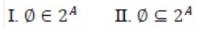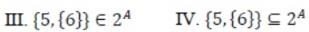Solution: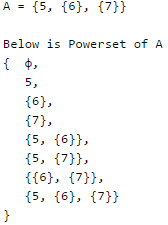I is true, as φ belongs to Powerset.
II is true, as an empty set is a subset of every set.
III is true as {5, {6}} belongs to Powerset.
IV is false, {5, {6}} is not a subset, but {{5, {6}}} is.

QUESTION: 14

The number of 4 digit numbers having their digits in non-decreasing order (from left to right) constructed by using the digits belonging to the set {1, 2, 3} is ____.

Solution:

{1, 1, 1, 1} {1, 1, 1, 2} {1, 1, 1, 3} {1, 1, 2, 2} {1, 1, 2, 3} {1, 1, 3, 3} {1, 2, 2, 2} {1, 2, 2, 3} {1, 2, 3, 3} {1, 3, 3, 3} {2, 2, 2, 2} {2, 2, 2, 3} {2, 2, 3, 3} {2, 3, 3, 3} {3, 3, 3, 3}

QUESTION: 15

How many substrings of different length (non-zero) can be formed from a character string of length n ?

Solution: